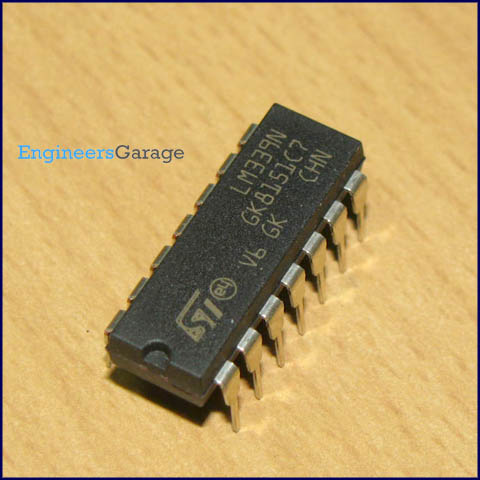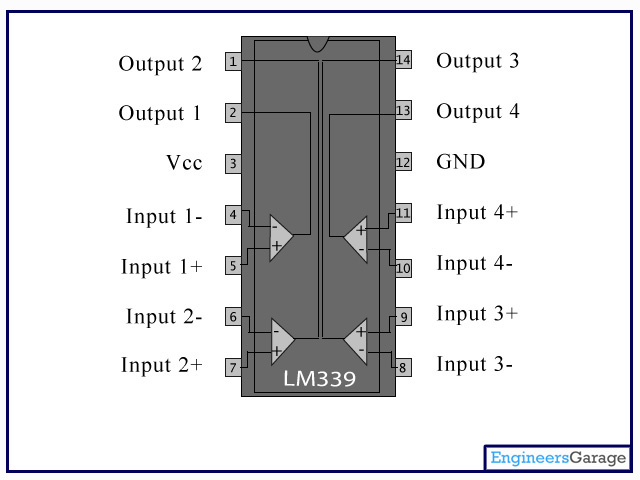﻿ LM339 | Comparator LM339 IC | Pin Diagram & Datasheet

# LM339 IC

Written By:

• Kushagra

### Image:### DataSheet:LM339.pdf

LM339 is a comparator IC with four inbuilt comparators. A comparator is a simple circuit that moves signals between the analog and digital worlds. It compares two input voltage levels and gives digital output to indicate the larger one. The two input pins are termed as inverting (V-) and non-inverting (V+). The output pin goes high when voltage at V+ is greater than that at V-, and vice versa. In common applications, one of the pins is provided with a reference voltage and the other one receives analog input from a sensor or any external device. If inverting pin (V-) is set as reference, then V+ must exceed this reference to result in high output. For inverted logic, the reference is set at V+ pin.

### Pin Diagram:### Pin Description:

 Pin No Function Name 1 Output of 2nd comparator Output 2 2 Output of 1st comparator Output 1 3 Supply voltage; 5V (+36 or ±18V) Vcc 4 Inverting input of 1st comparator Input 1- 5 Non-inverting input of 1st comparator Input 1+ 6 Inverting input of 1st comparator Input 2- 7 Non-inverting input of 2nd comparator Input 2+ 8 Inverting input of 3rd comparator Input 3- 9 Non-inverting input of 3rd comparator Input 3+ 10 Inverting input of 4th comparator Input 4- 11 Non-inverting input of 4th comparator Input 4+ 12 Ground (0V) Ground 13 Output of 4th comparator Output 4 14 Output of 3rd comparator Output 3